﻿ 细菌群体感应机制的动力学建模研究

# 细菌群体感应机制的动力学建模研究The Dynamical Modeling Studies of the Quorum Sensing Mechanism in Bacteria

Abstract: Quorum sensing is a signal exchange between bacteria, which can regulate many important bio-logical functions of bacteria by monitoring their population density. At present researchers study the quorum sensing mechanisms mainly with experiment or mathematical modeling. The quorum sensing signaling pathways of bacteria are extremely complex, but theoretically we can focus on the signaling network modeling of key proteins to quantitatively discuss the dynamical mechanisms of bacterial quorum sensing. This paper reviews the research progress of bacterial quorum sensing network modeling both in the wild bacteria and artificial synthesis bacteria, including Gramnegative bacteria, Grampositive bacteria and the bacteria which both have Gramnegative and Grampositive quorum sensing systems. The various dynamical modeling methods of the quorum sensing systems and their regulation mechanism are discussed in details. Open questions for future modeling are also suggested and prospected.

1. 细菌的群体感应

1.1. 革兰氏阴性菌的群体感应系统

1.2. 革兰氏阳性菌的群体感应系统Figure 1. The LuxI/LuxR quorum sensing system of Gramnegative bacteria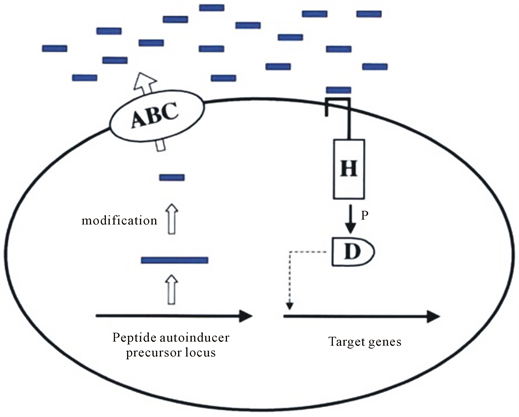Figure 2. Oligopeptide mediated quorum sensing system in Grampositive bacteria 

1.3. 革兰氏阳性菌和阴性菌共有的群体感应系统

20世纪90年代，在多个革兰氏阴性菌中发现了另外一套群体感应系统，它的群体感应自诱导因子由LuxS蛋白形成，称为自诱导因子2 (Autoinducer 2，AI-2)，其主要成分为呋喃酮酰硼酸二酯(Furanosyl borate diester)   。经磷酸化的调节子能够激活相关基因的转录，它可以参与革兰氏阳性和阴性菌的群体感应  ，一般认为这种信号分子是种间细胞交流的通用信号分子   。如在哈氏弧菌中，它的群体感应系统产生AHL类信号分子，但对它的检测却是一种类似革兰氏阳性细菌的双组份磷酸传递系统  。目前发现它还产生另外一种信号分子AI-2，AI-2分子的受体是LuxP蛋白  。LuxP-AI-2复合物结合到另一种蛋白LuxQ，LuxQ蛋白包含一个传感器激酶区和反应调节区     。当细菌密度低并且缺乏AI-2时，在LuxU中间蛋白的作用下，LuxQ将LuxO磷酸化。然后，磷酸化的LuxO激活了抑制荧光素酶操纵子转录的抑制蛋白的转录    。当细菌密度过高时，AI-2的出现，促使LuxQ磷酸化酶活性降低，从而使LuxO失活，这样导致LuxR介导荧光素酶的转录     。

1.4. 其他群体感应系统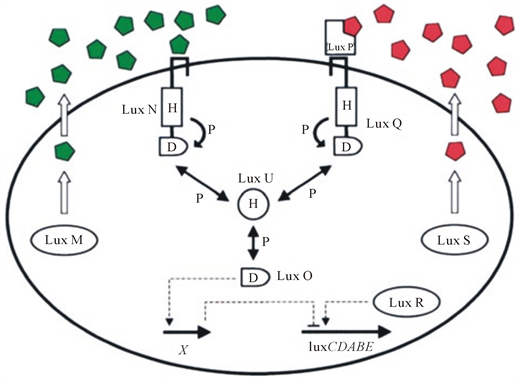Figure 3. The hybrid quorum sensing system circuit of V. harveyi 

2. 人工合成细菌的群体感应研究

2.1. 合成生物学的概念

1980年，德国科学家芭芭拉.荷本(Barbara Hobom)第一次开始用合成生物学这个学术名词来表述基因重组技术  。在工程学思想的指导下，合成生物学旨在利用测序技术、生物工程技术、化学合成技术、计算机建模及模拟技术等，从头设计并构建新的生物组件、设备和系统，或对现有的、天然的生物系统进行重新设计和改造，达到利用工程化的生物系统或生物模块来处理信息、制造材料、提供食物、操作化合物、生产能源、保持和增强人类健康及改善环境等目的    。传统的生物学是通过解剖生命体以研究其内在的构造，而合成生物学的研究方法完全是相反的，它是从最基本的要素开始逐步构建生物体的零部件直至人工生命系统  。

2.2. 人工合成细菌的群体感应系统实验研究介绍

You等  在2004年发表在Nature期刊上的文章重新构建了两个完全不同的基因片段，并把它们植入大肠杆菌细胞内，通过实验及模型证明了这两个片段可以互相耦合，并通过细胞交流的方式调控群体密度。

2006年，Li等  人工合成了大肠杆菌中AI-2群体信号分子的合成网络，通过哈氏弧菌中群体感应信号分子的模块来研究大肠杆菌中的AI-2群体信号分子的合成路径。得出了以下结论：1) 当细胞生长在葡萄糖存在的环境中，mRNA的转录和蛋白水平上AI-2的合成、Pfs和LuxS都不能显著提升AI-2的浓度；2) 在葡萄糖的存在下，这种合成途径伴随着代谢通量的增加，只是部分地解释了这种差异。他们预测，高通量的替代途径或其他生物步骤存在于AI-2的合成中。这些结果也得到了实验数据的验证。

2010年，Marguet等  构建了一个基因回路，它会引起细菌种群密度随时间的变化。基因回路含有两个模块，第一个模块赋予细胞杀死自身的作用，第二个模块是密度传感。他们的实验表明在含有合成自杀回路的大肠杆菌群体中产生的振荡不需要群体感应基因(luxR和luxI)，也不需要已知的PLuxI启动子中的调控元件。相反，振荡很可能是由于密度依赖质粒扩增建立的群体水平负反馈。

2013年，Saeidi等  人工合成了铜绿假单胞菌中的群体感应体系，并将其植入到大肠杆菌体内，通过测群体荧光强度来测量群体密度，他们的实验数据验证了他们建立的模型的正确性。

2013年，Daniel等  合成的模拟基因回路利用反馈来实现对数线性传感、比率和幂的计算。他们的回路可以被用来实现高阶函数，可以用复杂的生化模型和简单的数学函数描述。

2014年，Wang等  人工构建了LuxI/LuxR群体感应系统，并将其植入到大肠杆菌体内，通过调控细胞群体密度来研究LuxI/LuxR群体感应系统，对一系列标准化细胞浓度控制元件的工作性能进行了考察。实验结果表明，含有RBS0.07群体感应通路的工程菌能够对细胞浓度进行自我调整，相对于未含有该通路的菌株，该通路延长了菌体生长的稳定期。通过对含有不同效率RBS细胞浓度控制元件的菌株稳定期活菌数进行测定发现：RBS效率越高其稳定期活菌数越低。

2015年，Chen等  描述了一种由两类不同的细胞组成的合成微生物集团：“活化剂”菌株和“阻遏物”菌株。这些菌株产生两个正交的细胞信号分子，在两个菌株的合成回路内调节基因表达。这两种菌株只有在一起培养时才会产生明显的种群水平振荡。两种应变回路的某些网络拓扑结构比其他回路更好地保持鲁棒性。通过多个合作菌株的基因工程来规划种群水平动态的能力，为多种细胞类型中人工合成组织和器官的设计指明了方向。

2016年，Huang等  构建了一个信号网络来调控细菌的存活。工程菌在医学和环境方面有很大的应用潜力。实现工程菌的应用需要工程行为的可控性和工程系统的可伸缩性。在此，他们提出了一种平台技术，即微生物群，其采用空间布局来控制工程菌的生长动态。他们构建了几种合成基因回路来调控在大肠杆菌中的集体生存：只有在足够高的种群中，工程菌才能存活。他们将工程菌群封装成空心水凝胶微胶囊，作为微生物群的基本单元，从而实现种群的空间布局。当这些细菌被可渗透膜包裹时，能感应到膜内的环境并作出相应的反应。由于其较高的密度，渗透膜包裹的细胞将存活下来。然而，那些从渗透膜中逃脱的细菌，由于密度的降低，将会被杀死。他们证明了这个设计概念是模块化的，易于推广。他们的工作为混合生物材料系统的工程集成和可编程控制奠定了基础。

3. 群体感应研究中的数学模型

3.1. 革兰氏阴性菌中的群体感应模型

3.1.1. Dockery模型

2001年，基于已知的生物化学中对信号机制极其重要的自诱导因子的调节，Dockery等  针对铜绿假单胞菌中的群体感应系统建立了一个数学模型。Dockery等的模型中只考虑Las系统，信号网络见图4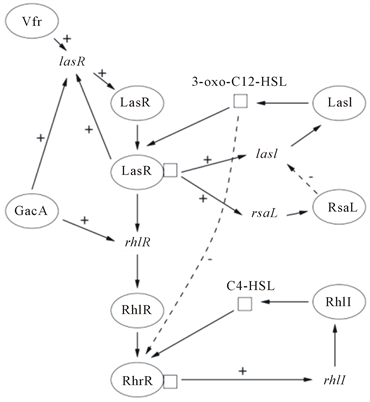Figure 4. Schematic diagram showing the gene regulation for the las and rhl systems in P. aeruginosa 

Dockery等建立了一个偏微分方程来研究细胞外空间的自诱导因子的非均匀分布，

$\begin{array}{l}\frac{\text{d}A}{\text{d}t}=F\left(A\right)+\frac{\delta }{\rho }\left(E-A\right)\\ \frac{\partial E}{\partial t}=\frac{{\partial }^{2}E}{\partial {x}^{2}}+\frac{\delta }{1-\rho }\left(A-E\right)-{k}_{E}E\\ {E}_{x}\left(L,t\right)+\alpha E\left(L,t\right)=0\\ {E}_{x}\left(0,t\right)=0\end{array}$ (3.1)

3.1.2. Chen模型

2004年，Chen等  以rhlI缺失突变株为研究对象，研究了自诱导因子PAI2对铜绿假单胞菌产生鼠李糖脂(RL)的影响。他们建立了一个模型来描述由rhl群体感应系统调控的RL生成动力学：首先，PAI2与RhlR蛋白结合；其次，激活的复合物RhlR:PAI2触发编码鼠李糖基转移酶的rhlAB操纵子的转录(和表达)；最后，该酶催化RL的合成。该模型较好地描述了实验结果。模型的预测如下：在加入PAI2后，诱导酶的合成速率最高。随着自诱导剂的降解，诱导酶的合成速率随时间的延长而减小。然而，酶的浓度仍在不断增加，直到在自体酶耗尽时到达最大值。PAI2的添加浓度越高，酶的初始合成速率越高，诱导的合成时间也越长。随着酶浓度的增加，RL的产率也随之增加，导致RL浓度的加速上升。当PAI2耗尽时，RL浓度的增加呈线性增长。根据所得到的最佳拟合模型参数也得出了以下结论：对于更加复杂的细胞内RhlR蛋白，需要1.61 μM PAI2，即野生型PAO1固定相培养中PAI2浓度的一半左右。另一方面，以其最大速率的一半激活鼠李糖基转移酶合成将需要39%的RLR与PAI2的结合。培养基中的最大RL产率为0.042 g/L h，完全诱导培养至少需要1.61 h才能将该酶合成到产生RL所需的水平。

3.1.3. Melke模型

2010年，Melke等用生长的微小菌落的细胞模型在单细胞水平上来研究群体感应机制  。他们发现菌落确实有一个细胞密度依赖的行为，并且高度依赖于局部细胞群集和群体进化空间的几何形状。他们分析了信号网络的两个正反馈回路，找到多稳区、展示群体感应机制取决于不同的模型参数。该信号网络如图5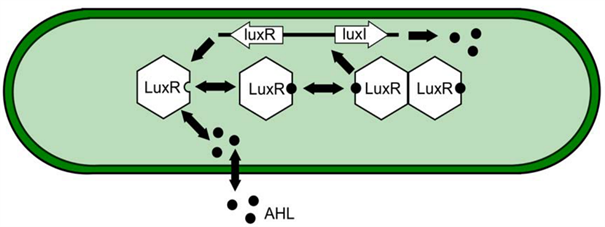Figure 5. The quorum sensing network in V. fischeri 

$\frac{\text{d}{A}_{e}^{i}}{\text{d}t}=\frac{D}{{v}_{e}}\underset{j\in {N}_{\left(i\right)}}{\sum }{a}_{ij}\frac{{A}_{e}^{j}-{A}_{e}^{i}}{{d}_{ij}}$

$\begin{array}{l}\frac{\text{d}A}{\text{d}t}=\frac{a}{{v}_{b}}{j}_{A}\\ \frac{\text{d}{A}_{e}}{\text{d}t}=-\frac{a}{{v}_{e}}{j}_{A}\\ {j}_{A}=-{p}_{in}A+{p}_{out}{A}_{e}.\end{array}$ (3.2)

Melke等发现，在细菌本身产生自诱导因子的自然环境中，相比于引入外部诱导因子的环境的交换能力，会导致更多的参数约束。以细胞为基础的模型也允许调查混合细胞群，在混合细胞群体中不产生诱导因子的细胞更具有适应性，但是在竞争中仍不能完全超越产生诱导因子的细胞。

3.1.4. Mcintosh模型

2013年，Mcintosh等  基于微分方程系统，对苜蓿根瘤菌中的群体感应建立了一个最小的数学模型，并对其做了随机模拟，随机模型中复合体ExpR/AHL的量不同于确定性模型，通过与确定性模型的对比表明该体系对于ExpR的总量是非常敏感的。该群体感应的参与因子有ExpR，SinR，SinI和该系统的信号分子AHL，信号网络图见图6

$\begin{array}{l}\frac{\text{d}{R}_{i}}{\text{d}t}={\alpha }_{1}\frac{1}{{\beta }_{3}{C}_{i}+1}-{\gamma }_{1}{R}_{i}\\ \frac{\text{d}{I}_{i}}{\text{d}t}={\alpha }_{2}\frac{{\beta }_{1}{R}_{i}}{{\beta }_{1}{R}_{i}+1}\left(1+{\alpha }_{2}\frac{{\beta }_{2}{C}_{i}}{{\beta }_{2}{C}_{i}+1}\right)-{\gamma }_{2}{I}_{i}\\ \frac{\text{d}{A}_{ii}}{\text{d}t}={\alpha }_{3}{I}_{i}-{\delta }_{1}{A}_{ii}+{\delta }_{2}{A}_{o}-{\alpha }_{4}\left(\stackrel{¯}{E}-{C}_{i}\right)-{\gamma }_{3}{A}_{ii}+{\gamma }_{4}{C}_{i}\\ \frac{\text{d}{A}_{O}}{\text{d}t}=B\left({\delta }_{1}{A}_{ii}-{\delta }_{2}{A}_{o}\right)-{\gamma }_{3}{A}_{O}\\ \frac{\text{d}{C}_{i}}{\text{d}t}={\alpha }_{4}{A}_{ii}\left(\stackrel{¯}{E}-{C}_{i}\right)-{\gamma }_{4}{C}_{i}.\end{array}$ (3.3)

Mcintosh等指出群体感应系统经历三个阶段：在细菌密度比较低时，所有产生的AHL迅速离开细胞；在中等细胞密度时，AHL的浓度增加的很缓慢，AHL的浓度随着细胞密度的增加而积累，AHL还可以被运回细胞去调节自身的生成等从而形成一个正反馈回路；如果细胞数相当多，AHL的生成就会关闭。

3.1.5. Barbarossa模型

2016年，Barbarossa 等  针对连续培养基中的恶臭假单胞菌中的群体感应系统提出了一种简单的时滞微分方程(Delay differential equations，简称DDES)，它是由于信号分子降解酶的作用产生了一个负反馈，从而有延迟而产生的时滞。这个群体感应系统包含一个正反馈和一(延迟)的负反馈机制。他们的研究结果展示了一些时滞微分方程解的基本性质，例如存在性、唯一性和非负性。最后一个性质对生物中的数学模型非常重要，在建立时滞微分方程时经常违背。研究了解的定性行为，特别是定态及其稳定性，结果表明，对于参数值的选择，系统对时滞给出稳定切换；另一方面，当时滞设为零时，可能会出现关于一个负反馈参数的霍普夫分歧。模型参数与实验数据吻合，表明时滞系统足以解释和预测生物观测值。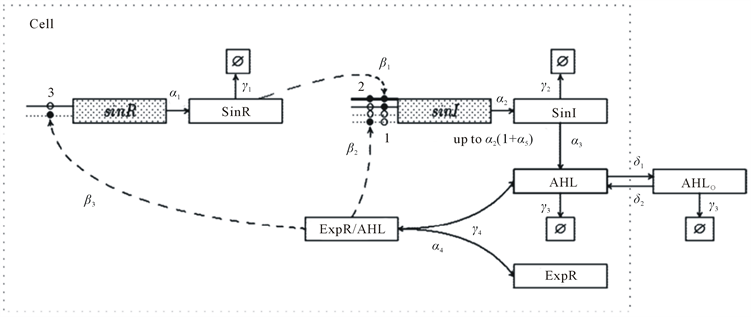Figure 6. The quorum sensing network in S. meliloti 

3.1.6. Marenda模型

$\frac{\partial C\left(x,t\right)}{\partial t}=\alpha {\rho }_{P}+{D}_{s}\frac{{\partial }^{2}C\left(x,t\right)}{\partial {x}^{2}}-{k}_{b}\left({\rho }_{P}\right)C\left(x,t\right)-{k}_{b}\left({\rho }_{R}\right)C\left(x,t\right)-{k}_{e}C\left(x,t\right)$ (3.4)

Ds是琼脂内信号分子的扩散常数，ke是细胞外环境中的化学降解速率， ${k}_{b}\left(\rho \right)$ 是生物降解速率，假设这些参数对于生产和报告菌株是一样的。为了满足边界条件，他们假设

$\begin{array}{l}{\partial C\left(x,t\right)|}_{x=0}=0\\ {\frac{\partial C\left(x,t\right)}{\partial x}|}_{x=h}=0\end{array}$ (3.5)

$f\left(C\left(x,t\right)\right)=\frac{C{\left(x,t\right)}^{m}}{C{\left(x,t\right)}^{m}+{C}^{\text{*}m}}$ (3.6)

$E+S\underset{{k}_{-1}}{\overset{{k}_{1}}{⇄}}ES\stackrel{{k}_{2}}{\to }E+P$ (3.7)

$\begin{array}{l}\frac{\partial \left[E\right]}{\partial t}=Af\left(x,t\right)-{k}_{1}\left[S\right]\left[E\right]+{k}_{-1}\left[ES\right]+{k}_{2}\left[ES\right]\\ \frac{\partial \left[S\right]}{\partial t}=-{k}_{1}\left[S\right]\left[E\right]+{k}_{-1}\left[ES\right]+{D}_{x}\frac{{\partial }^{2}\left[S\right]}{\partial {x}^{2}}\\ \frac{\partial \left[ES\right]}{\partial t}={k}_{1}\left[S\right]\left[E\right]-{k}_{-1}\left[ES\right]-{k}_{2}\left[ES\right]\\ \frac{\partial \left[P\right]}{\partial t}={k}_{2}\left[ES\right]+{D}_{x}\frac{{\partial }^{2}\left[P\right]}{\partial {x}^{2}}\end{array}$ (3.8)

3.2. 革兰氏阳性菌中的群体感应模型

3.2.1. Karlsson模型

$\begin{array}{l}\frac{\text{d}{X}_{ComD}}{\text{d}t}=\beta Com{D}^{n}+{v}_{\mathrm{max}}Com{D}^{n}\left(\frac{{X}_{ComE\text{~}P}}{{X}_{ComE\text{~}P+n{v}_{\mathrm{int}}kComD}}\right)-{\delta }_{ComD}{X}_{ComD}-\alpha {X}_{ComD}CSP+\gamma {X}_{ComDP}\\ \frac{\text{d}{X}_{ComDP}}{\text{d}t}=\alpha {X}_{ComD}CSP-\gamma {X}_{ComDP}-{\delta }_{ComDP}{X}_{ComDP}\\ \frac{\text{d}{X}_{ComE}}{\text{d}t}=\beta Com{E}^{n}+{v}_{\mathrm{max}}Com{E}^{n}\left(\frac{{X}_{ComE\text{~}P}}{{X}_{ComE\text{~}P+n{v}_{\mathrm{int}}kComE}}\right)-{\delta }_{ComE}{X}_{ComE}-\frac{\lambda }{n{v}_{\mathrm{int}}}{X}_{ComE}{X}_{ComDP}+\rho {X}_{ComE\text{~}P}\\ \frac{\text{d}{X}_{ComE\text{~}P}}{\text{d}t}=\frac{\lambda }{n{v}_{\mathrm{int}}}{X}_{ComE}{X}_{ComDP}-\rho {X}_{ComE\text{~}P}-{\delta }_{ComE\text{~}P}{X}_{ComE\text{~}P}\end{array}$ (3.9)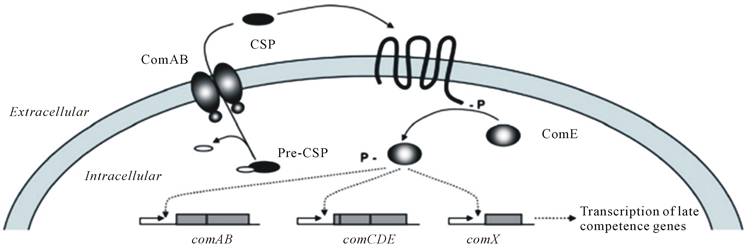Figure 7. Illustration of the quorum sensing regulated ComABCDE pathway in S. pneumonia 

3.2.2. Koerber模型

AgrD通过AgrB的处理生成AIP，生成的AIP可以被分泌到细胞外。AgrC是AIP的接受体，并使AgrA磷酸化，然后与SarA作用促进启动子P2和P3调节ageBDCA和RNAIII的表达  。

3.3. 革兰氏阳性菌和阴性菌的共有的群体感应模型

3.3.1. Banik模型

2009年，Banik等  建立了一个简单的模型来研究信号分子在群体感应中传导的通路，分析了控制系统反应的关键的无量纲参数。这些参数是利用不同突变株的发光表型的实验数据来估计的。相应的模型预测与不作为确定参数输入的实验结果相一致。此外，提出的框架可以预测发光表型的变化和网络结构的扰动。对于信号分子绑定到它的同源受体上的过程，一般用如下动力学反应方程来表示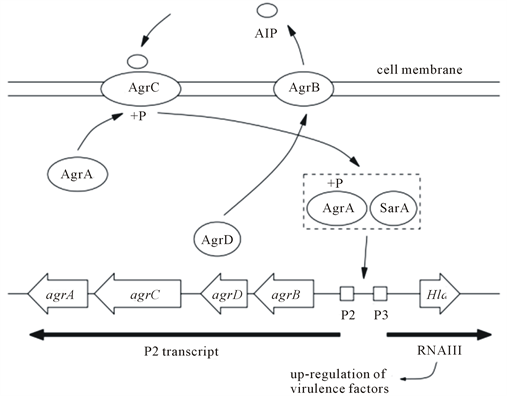Figure 8. A schematic of the proposed agr system in S. aureus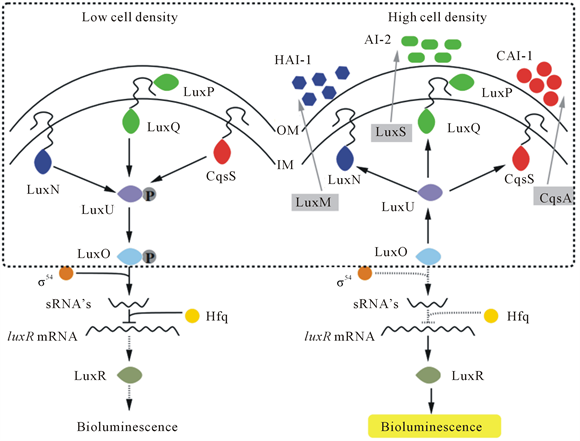Figure 9. The V. harveyi quorum sensing gene network 

${S}_{ki}+A{I}_{i}\underset{{k}_{-1}}{\overset{{k}_{1}}{⇄}}{S}_{pi}$ (3.10)

$\left[{S}_{ki}\right]=\left(1-{g}_{i}\right){c}_{i}\left[{S}_{0}\right]\left[{S}_{pi}\right]={g}_{i}{c}_{i}\left[{S}_{0}\right]$ (3.11)

$\begin{array}{l}\left[{S}_{ki}\right]+\left[{S}_{pi}\right]={c}_{i}\left[{S}_{0}\right]\left[{S}_{pi}\right]={g}_{i}{c}_{i}\left[{S}_{0}\right]\\ {g}_{i}={a}_{i}^{n}/\left(1+{a}_{i}^{n}\right)\\ {a}_{i}=\left[A{I}_{i}\right]/{\kappa }_{i}\\ {\kappa }_{i}={k}_{-i}/{\kappa }_{i}\end{array}$ (3.12)

3.3.2. 其他模型

3.4. 人工构建的群体感应系统模型

3.4.1. Nilsson模型

1983年，Lee等从基因层面利用分子机理模型分析了生长速率对重组大肠杆菌种群生产力的影响  。2001年，Nilsson等  建立了一个数学模型来模拟细胞内和膜上的信号分子AHL的浓度随时间的变化。他们的模型如下：

$\begin{array}{l}\frac{\text{d}{\eta }_{bc}}{\text{d}t}=h\left({C}_{bc}\right){N}_{\left(t\right)}-{\text{Δ}}_{bf}\\ \frac{\text{d}{\eta }_{bf}}{\text{d}t}={\text{Δ}}_{bc}-{\text{Δ}}_{bf}\\ N\left(t\right)=\frac{K}{1+\left(\frac{K}{{N}_{0}}-1\right){e}^{-\rho t}}\\ {\text{Δ}}_{bf}=\alpha {A}_{bc}\left({C}_{bf}-{C}_{bc}\right)\\ {C}_{bc}=\frac{{\eta }_{bc}}{\frac{4\pi {r}_{bc}^{3}}{3}N\left(t\right)}\\ {C}_{bf}=\frac{{\eta }_{bf}}{{V}_{\mathrm{int}}}\\ {V}_{\mathrm{int}}={V}_{bf}-{V}_{bc}\end{array}$ (3.13)

${\eta }_{bc}$ 是细胞内AHL的总量， ${\eta }_{bf}$ 是细胞膜内AHL的总量。 $4\pi {r}_{bc}^{3}/3$ 代表所有细胞的总体积， $h\left({C}_{bc}\right)$ 表示AHL的净产率， ${N}_{\left(t\right)}$ 表示以时间以t为函数的细胞数， ${\text{Δ}}_{bc}$ 表示AHL扩散出细胞内的净扩散速率， ${\text{Δ}}_{bf}$ 表示AHL扩散出细菌细胞膜外的净扩散速率。K (0 < K)对应于细菌达到渐近细胞密度时的细菌密度(例如“固定相”)的密度 $N\left(K\right)$${N}_{0}$ 是种群中细菌的初始密度，而 $\rho$ (0 < $\rho$ ) 是内在增长率， $\rho$ 是一个种群参数，因此是整个细菌种群中细胞分裂率的平均值。 $\alpha$ 是通透性常数， ${A}_{bc}$ 是单个细菌的表面积， ${C}_{bf}$ 是细胞膜内AHL的浓度， ${C}_{bc}$ 是细菌内的AHL的浓度， ${V}_{\mathrm{int}}$ 是细胞膜的总体积， ${V}_{bf}$ 是细菌的总体积， ${V}_{bc}$ 是细胞内的总体积， $\gamma$ 是决定细胞密度的一个参数。

AHL浓度随时间的改变可以反映群体生长速率、AHL扩散速率和自身诱导速率。Nilsson等模拟的结果显示单个细菌内的信号分子浓度在细菌生长初始阶段会成倍增加，接着达到一个稳定的平衡期，然后会有一个下降阶段，接着达到第二个稳定平衡期。开始细胞内部高浓度的AHL积累是由于信号分子扩散到细胞外的速率低，细菌生长速率低，自诱导因子分泌速率高。相反的，平衡稳定期时，细菌生长速率高，自诱导因子分泌速率低，信号分子扩散到细胞外的速率高。这些结果表明AHL介导的表达可以在相对较低的细胞密度和低水平的外部环境中的AHL浓度下发生。

3.4.2. You模型

2003年，Ojalvo等  利用群体感应耦合模拟了一种合成的多细胞时钟。Marguet等  用数学模型分析了怎么通过扩散损耗减少群体感应里的噪声。

You等  在2004年发表在Nature期刊上的文章通过模型证明了luxI/luxR 基因与目的基因这两个片段可以互相耦合，并通过细胞交流的方式调控群体密度。You等合成的群体感应网络见图10，模型如下：

$\frac{\text{d}N}{\text{d}t}=k\text{N}\left(1-N/{N}_{m}\right)-\text{d}EN$ (3.14)

$\frac{\text{d}E}{\text{d}t}={k}_{E}\text{A}-{d}_{E}\text{E}$ (3.15)

$\frac{\text{d}A}{\text{d}t}={v}_{A}\text{N}-{d}_{A}\text{A}$ (3.16)

1) 作者构建的系统在合适的参数值下可以达到稳定的菌群密度。

2) 在植入合成基因片段的体系中，致死蛋白的生成速率受信号分子合成的制约。

3) 稳定时的菌体密度和信号分子的降解速率常数成比例。

4) 当环境中的pH改变时，细胞内的致死蛋白的量保持稳定。Figure 10. A population-control circuit programmes population dynamics by broadcasting, sensing and regulating the cell density using cell-cell communication and negative feedback 

3.4.3. Chen模型

Chen等  在2005年提供了一个通用的模型和分析工具，用来分析细胞内和细胞外的随机波动对多系统的合作行为。他们建模时都有考虑细胞外环境中的信号分子，分析了信号在细胞间传递的机制。下面我们以Chen等的模型详细介绍建立模型研究群体感应机制时怎么考虑信号分子从细胞内流出细胞外的过程。

Chen等的工作将信号分子的扩散也考虑进模型中，进一步分析了群体感应机制的作用机理，他们用合成基因网络构成的多系统来证明噪声、延迟和耦合对集体动力学的影响。这些结果对理解噪音在合作动力学中的重要作用奠定了理论和定量基础，例如细胞间的交流。他们构建的信号网络见图11

$\begin{array}{l}LuxI\stackrel{{k}_{a}}{\to }LuxI+AI\\ AI+AI\underset{{k}_{-1}}{\overset{{k}_{1}}{⇄}}A{I}_{2}\\ LuxR+LuxR\underset{{k}_{-2}}{\overset{{k}_{2}}{⇄}}Lux{R}_{2}\\ A{I}_{2}+Lux{R}_{2}\underset{{k}_{-3}}{\overset{{k}_{3}}{⇄}}AL\\ AL+DNA\underset{{k}_{-4}}{\overset{{k}_{4}}{⇄}}ALD\\ DNA\stackrel{{k}_{m}}{\to }mRN{A}_{LuxI}+mRN{A}_{LuxR}+DNA\end{array}$ (3.17)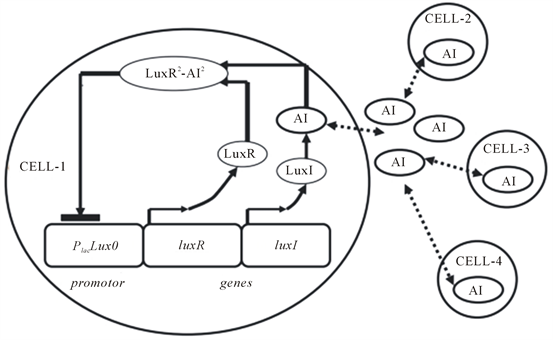Figure 11. A two-gene model of a gene regulatory network 

$\begin{array}{l}ALD\stackrel{\alpha {k}_{m}}{\to }mRN{A}_{LuxI}+mRN{A}_{LuxR}+ALD\\ mRN{A}_{LuxI}\stackrel{{k}_{pi}}{\to }LuxI+mRN{A}_{LuxI}\\ mRN{A}_{LuxR}\stackrel{{k}_{pr}}{\to }LuxI+mRN{A}_{LuxR}\end{array}$ (3.18)

$AI\underset{d\upsilon /V}{\overset{d}{⇄}}Y\text{withfeedbackdelay}\tau$ (3.19)

$AI\underset{{\sigma }^{2}\upsilon /\left(2Y\right)}{\overset{{\sigma }^{2}\upsilon /\left(2AI\right)}{⇄}}Y$ (3.20)

3.4.4. Li模型

2006年，Li等  为了研究大肠杆菌中的AI-2信号通路，建立了一个随机模型。对于生物系统，若具有非常低的物种浓度(或数量)和缓慢的反应速率，由于固有的随机事件，随机波动可能存在。反过来也可以产生显著的系统行为变异，特别是随机建模时。由于AI-2的浓度非常低，所以Li等建立了一个随机模型来研究AI-2信号通路。

Li等通过研究AI-2的合成、提取和调控，提出了一些可检测的假设，得出了以下结论：1) 当细胞生长在葡萄糖存在的环境中，mRNA的转录和蛋白水平上AI-2的合成、Pfs和LuxS都不能显著提升AI-2的浓度；2) 在葡萄糖的存在下，这种合成途径伴随着代谢通量的增加，只是部分地解释了这种差异。他们预测，高通量的替代途径或其他生物步骤存在于AI-2的合成中；3) 实验结果验证了这一假说。这项工作证实了基于系统的随机模型可以和细胞生理学联系起来。Tian等也有用随机模型来研究群体感应  。

3.4.5. Saeidi模型

2013年，Saeidi等  人工合成了一个群体感应系统，针对人工合成的群体感应体系来研究铜绿假单胞菌中的群体感应机制，他们的模型从基因层面上更加详细地对细菌群体感应机制进行了模拟。下面我们以Saeidi等的模型为例详细讲解从基因层面建模研究群体感应的过程，他们人工构建的网络如图12所示。

$\frac{\text{d}\left[mRN{A}_{LasR}\right]}{\text{d}t}={k}_{1}\left[\text{pTetR}\right]-{y}_{1}\left[mRN{A}_{LasR}\right]$ (3.21)

$\frac{\text{d}\left[LasR\right]}{\text{d}t}={k}_{2}\left[mRN{A}_{LasR}\right]-{y}_{2}\left[LasR\right]$ (3.22)

$\frac{\text{d}\left[LasR-AHL\right]}{\text{d}t}={k}_{1}\left[LasR\right]\left[AHL\right]-{k}_{4}\left[LasR-AHL\right]$ (3.23)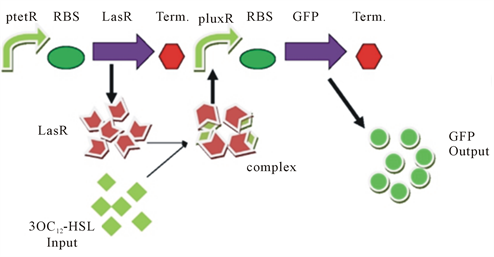Figure 12. A schematic of the quorum sensing system modeled 

$\frac{d\left[AHL\right]}{dt}=-{k}_{3}\left[LasR\right]\left[AHL\right]+{k}_{4}\left[LasR-AHL\right]$ (3.24)

$\frac{\text{d}\left[A.pLuxR\right]}{\text{d}t}={k}_{5}\left[LasR-AHL\right]\left[pLuxR\right]-{k}_{6}\left[A.pLuxR\right]$ (3.25)

$\frac{\text{d}\left[GFP\right]}{\text{d}t}=\frac{1}{T}\left(GF{P}_{\mathrm{max}}-\left[GFP\right]\right)$ (3.26)

$\frac{1}{T}={k}_{7}+\frac{{k}_{8}{\left[A.pLuxR\right]}^{{n}_{1}}}{{k}_{9}^{{n}_{1}}+{\left[A.pLuxR\right]}^{{n}_{1}}}$ (3.27)

$\frac{1}{T}={k}_{10}+\frac{{k}_{11}{\left[A.pLuxR\right]}^{{n}_{2}}}{{k}_{12}^{{n}_{2}}+{\left[A.pLuxR\right]}^{{n}_{2}}}$ (3.28)

4. 总结与展望

1) 构建的信号通路可能还不够完善，群体感应机制中更加细分的许多过程还不明确，群体感应系统中涉及到的重要蛋白组分在细胞中也可能参与到其他生命活动中。随着人们对群体感应系统研究的深入，如果能将更多的生命活动的信号网络和群体感应系统的信号网络联系在一起，逐渐完善细胞生命活动的信号网络，那么人们对群体感应系统的研究乃至细胞整个生命活动的研究都会更加深入和细致，便于人们对生物体生命活动进行进一步地了解。

2) 现在人们构建的群体感应模型中虽然有些是从基因层面开始构建的，但是具体的诱导剂作用到基因上的启动子，启动子启动基因的转录翻译这些过程人们都还不是很明确，在构建模型时一般都只省略概括为一到两个方程，这样的模型并没有将生物体真实的生命活动完全还原出来，对细菌群体感应系统及生物体的真实生命活动过程的研究都是不足的，在今后的研究中，构建数学模型时，这些过程都要更加详细地表示出来，更加真实地还原生命活动。

3) 目前人们在研究群体感应系统时构建的数学模型对细胞的死亡过程考虑的还不是很全面，对于细胞生长后期，培养体系中营养物质缺乏时，细胞由于代谢副产物的积累，具体是哪些副产物导致细胞死亡的机制不是特别明晰，因此在建立数学模型时不能很好地遵循真实生命活动来建模，今后人们在研究群体感应系统建模时要更加明确细胞生长后期细胞自身的死亡机制，更接近于真实生命活动来建立数学模型。

4) 现在人们在研究细菌群体感应系统建模时建立的数学模型一般都是简单的常微分方程，而且忽略了许多生命过程中的噪声及空间扩散、边界效应的影响，这样建立的数学模型可能并不能真实地反应生命活动，因此在今后建立数学模型时，要将每个生命活动的机制都研究清楚，有些不能忽略的噪声、信号分子之间的相互作用、空间扩散、边界效应等都要考虑在内，在更接近真实情况下准确地建立数学模型。遇到具有非常低浓度(或数量)的物种的遗传调控网络和缓慢的反应速率，由于固有的随机事件，随机波动可能存在，这种情况下可以建立随机模型。

NOTES

*通讯作者。

 Parsek, M.L. Val, D., Hanzelka, B., Cronan, J. and Greenberg, E.P. (1999) Acyl Homoserine-Lactone Quorum-Sensing Signal Generation. Proceedings of the National Academy of Sciences of the United States of America, 96, 8789-8793.
https://doi.org/10.1073/pnas.96.8.4360

 Dandekar, A.A., Chugani, S. and Greenberg, E.P. (2012) Bacterial Quorum Sensing and Metabolic Incentives to Cooperate. Science, 338, 264-266.
https://doi.org/10.1126/science.1227289

 Li, Y.H., Tang, N., Aspiras, M.B., Lau, P.C.Y., Lee, J.H., Ellen, R.P. and Cvitkovitch, D.G. (2002) A Quorum-Sensing Signaling System Essential for Genetic Competence in Streptococcus mutans Is Involved in Biofilm Formation. Journal of Bacteriology, 184, 2699-2708.
https://doi.org/10.1128/JB.184.10.2699-2708.2002

 Diggle, S.P., Griffin, A.S., Campbell, G.S. and West, S.A. (2007) Cooperation and Conflict in Quorum-Sensing Bacterial Populations. Nature, 450, 411-414.
https://doi.org/10.1038/nature06279

 Hooshangi, S. and Bentley, W.E. (2008) From Unicellular Properties to Multicellular Behavior: Bacteria Quorum Sensing Circuitry and Applications. Current Opinion in Biotechnology, 19, 550-555.
https://doi.org/10.1016/j.copbio.2008.10.007

 Stoodley, P., Sauer, K., Davies, D.G. and Costerton, J.W. (2002) Biofilms as Complex Differentiated Communities. Annual Review of Microbiology, 56, 187-209.
https://doi.org/10.1146/annurev.micro.56.012302.160705

 Hagen, S.J., Son, M., Weiss, J.T. and Young, J.H. (2010) Bacterium in a Box: Sensing of Quorum and Environment by the LuxI/LuxR Gene Regulatory Circuit. Journal of Biological Physics, 36, 317-327.
https://doi.org/10.1007/s10867-010-9186-4

 Ng, W.L. and Bassler, B.L. (2009) Bacterial Quorum-Sensing Network Architectures. Annual Review of Genetics, 43, 197-222.
https://doi.org/10.1146/annurev-genet-102108-134304

 Tsai, C.S. and Winans, S.C. (2010) LuxR-Type Quo-rum-Sensing Regulators That Are Detached from Common Scents. Molecular Microbiology, 77, 1072-1082.
https://doi.org/10.1111/j.1365-2958.2010.07279.x

 Daniels, R., Vanderleyden, J. and Michiels, J. (2004) Quorum Sensing and Swarming Migration in Bacteria. FEMS Microbiology Reviews, 28, 261-289.
https://doi.org/10.1016/j.femsre.2003.09.004

 Hao, Y., Winans, S.C., Glick, B.R. and Charles, T.C. (2010) Identification and Characterization of New LuxR/LuxI-Type Quorum Sensing Systems from Metagenomic Libraries. Environmental Microbiology, 12, 105-117.
https://doi.org/10.1111/j.1462-2920.2009.02049.x

 Kambam, P.K., Sayut, D.J., Niu, Y., Eriksen, D.T. and Sun, L. (2008) Directed Evolution of LuxI for Enhanced OHHL Production. Biotechnology and Bioengineering, 101, 263-272.
https://doi.org/10.1002/bit.21901

 Rampioni, G., Leoni, L. and Williams, P. (2014) The Art of Antibacterial Warfare: Deception through Interference with Quorum Sensing-Mediated Communication. Bioorganic Chemistry, 55, 60-68.
https://doi.org/10.1016/j.bioorg.2014.04.005

 Steindler, L. and Venturi, V. (2007) Detection of Quorum-Sensing N-Acyl Homoserine Lactone Signal Molecules by Bacterial Biosensors. FEMS Microbiology Letters, 266, 1-9.
https://doi.org/10.1111/j.1574-6968.2006.00501.x

 Li, Z. and Nair, S.K. (2012) Quorum Sensing: How Bacteria Can Coordinate Activity and Synchronize Their Response to External Signals? Protein Science, 21, 1403-1417.
https://doi.org/10.1002/pro.2132

 Jayaraman, A. and Wood, T.K. (2008) Bacterial Quorum Sensing: Signals, Circuits, and Implications for Biofilms and Disease. Annual Review of Biomedical Engineering, 10, 145-167.
https://doi.org/10.1146/annurev.bioeng.10.061807.160536

 Bjarnsholt, T., Jensen, P.O., Jakobsen, T.H., Phipps, R., Nielsen, A.K., Rybtke, M.T., Tolker-Nielsen, T., Givskov, M., Hoiby, N. and Ciofu, O., The Scandinavian Cystic Fibrosis Study Consortium (2010) Quorum Sensing and Virulence of Pseudomonas aeruginosa during Lung Infection of Cystic Fibrosis Patients. PLoS ONE, 5, e10115.
https://doi.org/10.1371/journal.pone.0010115

 Whitehead, N.A., Barnard, A.M.L., Slater, H., Simpson, N.J.L. and Salmond, G.P.C. (2001) Quorum-Sensing in Gram-Negative Bacteria. FEMS Microbiology Reviews, 25, 365-404.

 Perez, P.D. and Hagen, S.J. (2010) Heterogeneous Response to a Quorum-Sensing Signal in the Luminescence of Individual Vibrio fischeri. PLoS ONE, 5, e15473.
https://doi.org/10.1371/journal.pone.0015473

 Scott, S.R. and Hasty, J. (2016) Quorum Sensing Communication Modules for Microbial Consortia. ACS Synthetic Biology, 5, 969-977.
https://doi.org/10.1021/acssynbio.5b00286

 Marchand, N. and Collins, C.H. (2016) Synthetic Quorum Sensing and Cell-Cell Communication in Gram-Positive Bacillus megaterium. ACS Synthetic Biology, 5, 597-606.
https://doi.org/10.1021/acssynbio.5b00099

 Davis, R.M., Muller, R.Y. and Haynes, K.A. (2015) Can the Natural Diversity of Quorum-Sensing Advance Synthetic Biology? Frontiers in Bioengineering and Biotechnology, 3, 30.

 Annous, B.A., Fratamico, P.M. and Smith, J.L. (2009) Scientific Status Summary. Journal of Food Science, 74, R24-R37.
https://doi.org/10.1111/j.1750-3841.2008.01022.x

 Antunes, L.C., Ferreira, R.B., Buckner, M.M. and Finlay, B.B. (2010) Quorum Sensing in Bacterial Virulence. Microbiology, 156, 2271-2282.
https://doi.org/10.1099/mic.0.038794-0

 Rutherford, S.T. and Bassler, B.L. (2012) Bacterial Quorum Sensing: Its Role in Virulence and Possibilities for Its Control. Cold Spring Harbor Perspectives in Medicine, 2, 705-709.
https://doi.org/10.1101/cshperspect.a012427

 Deep, A., Chaudhary, U. and Gupta, V. (2011) Quorum Sensing and Bacterial Pathogenicity: From Molecules to Disease. Journal of Laboratory Physicians, 3, 4-11.
https://doi.org/10.4103/0974-2727.78553

 Novick, R.P. (2003) Autoinduction and Signal Transduction in the Regulation of Staphylococcal Virulence. Molecular Microbiology, 48, 1429-1449.
https://doi.org/10.1046/j.1365-2958.2003.03526.x

 Defoirdt, T., Miyamoto, C.M., Wood, T.K., Meighen, E.A., Sorgeloos, P., Verstraete, W. and Bossier, P. (2007) The Natural Furanone (5Z)-4-Bromo-5-(Bromomethylene)-3-Butyl-2(5H)-Furanone Disrupts Quorum Sensing-Regulated Gene Expression in Vibrio harveyi by Decreasing the DNA-Binding Activity of the Transcriptional Regulator Protein LuxR. Environmental Microbiology, 9, 2486-2495.
https://doi.org/10.1111/j.1462-2920.2007.01367.x

 Fernandes, R., Roy, V., Wu, H.C. and Bentley, W.E. (2010) Engineered Biological Nanofactories Trigger Quorum Sensing Response in Targeted Bacteria. Nature Nanotechnology, 5, 213-217.
https://doi.org/10.1038/nnano.2009.457

 Abubakar, B.M. (2014) The Significant Role of Quorum Sensing in the Control of Vibrio cholerae Virulence. Advances in Bioscience and Bioengineering, 2, 1.

 Camilli, A. and Bassler, B.L. (2006) Bacterial Small-Molecule Signaling Pathways. Science, 311, 1113-1116.
https://doi.org/10.1126/science.1121357

 Ahlgren, N.A., Harwood, C.S., Schaefer, A.L., Giraud, E. and Greenberg, E.P. (2011) Aryl-Homoserine Lactone Quorum Sensing in Stem-Nodulating Photosynthetic Bradyrhizobia. Proceedings of the National Academy of Sciences of the United States of America, 108, 7183-7188.
https://doi.org/10.1073/pnas.1103821108

 Venturi, V., Rampioni, G., Pongor, S. and Leoni, L. (2011) The Virtue of Temperance: Built-In Negative Regulators of Quorum Sensing in Pseudomonas. Molecular Microbiology, 82, 1060-1070.
https://doi.org/10.1111/j.1365-2958.2011.07890.x

 Xavier, K.B. and Bassler, B.L. (2005) Interference with AI-2-Mediated Bacterial Cell-Cell Communication. Nature, 437, 750-753.
https://doi.org/10.1038/nature03960

 Swords, W.E. (2012) Quorum Signaling and Sensing by Nontypeable Haemophilus influenzae. Frontiers in Cellular and Infection Microbiology, 2, 100.
https://doi.org/10.3389/fcimb.2012.00100

 Papenfort, K. and Bassler, B.L. (2016) Quorum Sensing Sig-nal-Response Systems in Gram-Negative Bacteria. Nature Reviews Microbiology, 14, 576-588.
https://doi.org/10.1038/nrmicro.2016.89

 von Bodman, S.B., Willey, J.M. and Diggle, S.P. (2008) Cell-Cell Communication in Bacteria: United We Stand. Journal of Bacteriology, 190, 4377-4391.
https://doi.org/10.1128/JB.00486-08

 Collins, C.H., Leadbetter, J.R. and Arnold, F.H. (2006) Dual Selection Enhances the Signaling Specificity of a Variant of the Quorum-Sensing Transcriptional Activator LuxR. Nature Biotechnology, 24, 708-712.
https://doi.org/10.1038/nbt1209

 Neiditch, M.B., Federle, M.J., Miller, S.T., Bassler, B.L. and Hughson, F.M. (2005) Regulation of LuxPQ Receptor Activity by the Quorum-Sensing Signal Autoinducer-2. Molecular Cell, 18, 507-518.

 Pappas, K.M., Weingart, C.L. and Winans, S.C. (2004) Chemical Communication in Proteobacteria: Biochemical and Structural Studies of Signal Synthases and Receptors Required for Intercellular Signalling. Molecular Microbiology, 53, 755-769.
https://doi.org/10.1111/j.1365-2958.2004.04212.x

 Rutherford, S.T., van Kessel, J.C., Shao, Y. and Bassler, B.L. (2011) Apha and LuxR/HapR Reciprocally Control Quorum Sensing in Vibrios. Genes & Development, 25, 397-408.

 Heinemann, M. and Panke, S. (2006) Synthetic Biology—Putting Engineering into Biology. Bioinformatics, 22, 2790-2799.
https://doi.org/10.1093/bioinformatics/btl469

 Leonard, E., Nielsen, D., Solomon, K. and Prather, K.J. (2008) Engineering Microbes with Synthetic Biology Frameworks. Trends in Biotechnology, 26, 674-681.

 Swami, M. (2009) Synthetic Biology: Avoiding the Chop. Nature Reviews Genetics, 10, 660-660.
https://doi.org/10.1038/nrg2671

 Bedau, M.A., Parke, E.C., Tangen, U. and Hantsche-Tangen, B. (2009) Social and Ethical Checkpoints for Bottom-Up Synthetic Biology, or Protocells. Systems and Synthetic Biology, 3, 65-75.
https://doi.org/10.1007/s11693-009-9039-2

 Danino, T., Mondragon-Palomino, O., Tsimring, L. and Hasty, J. (2010) A Synchronized Quorum of Genetic Clocks. Nature, 463, 326-330.
https://doi.org/10.1038/nature08753

 Haseltine, E.L. and Arnold, F.H. (2008) Implications of Rewiring Bacterial Quorum Sensing. Applied and Environmental Microbiology, 74, 437-445.
https://doi.org/10.1128/AEM.01688-07

 Webster, S.M., Del Camino, D., Dekker, J.P. and Yellen, G. (2004) Intracellular Gate Opening in Shaker K+ Channels Defined by High-Affinity Metal Bridges. Nature, 428, 864-868.
https://doi.org/10.1038/nature02468

 Li, J., Wang, L., Hashimoto, Y., Tsao, C.Y., Wood, T.K., Valdes, J.J., Zafiriou, E. and Bentley, W.E. (2006) A Stochastic Model of Escherichia coli AI-2 Quorum Signal Circuit Reveals Alternative Synthesis Pathways. Molecular Systems Biology, 2, 67.
https://doi.org/10.1038/msb4100107

 Saeidi, N., Arshath, M., Chang, M.W. and Poh, C.L. (2013) Characterization of a Quorum Sensing Device for Synthetic Biology Design: Experimental and Modeling Validation. Chemical Engineering Science, 103, 91-99.
https://doi.org/10.1016/j.ces.2012.12.016

 Daniel, R., Rubens, J.R., Sarpeshkar, R. and Lu, T.K. (2013) Synthetic Analog Computation in Living Cells. Nature, 497, 619-623.
https://doi.org/10.1038/nature12148

 Wang, Z., Wu, X., Peng, J., Hu, Y., Fang, B. and Huang, S. (2014) Artificially Constructed Quorum-Sensing Circuits Are Used for Subtle Control of Bacterial Population Density. PLoS ONE, 9, e104578.
https://doi.org/10.1371/journal.pone.0104578

 Huang, S., Lee, A.J., Tsoi, R., Wu, F., Zhang, Y., Leong, K.W. and You, L. (2016) Coupling Spatial Segregation with Synthetic Circuits to Control Bacterial Survival. Molecular Systems Biology, 12, 859.
https://doi.org/10.15252/msb.20156567

 Dockery, J.D. and Keener, J.P. (2001) A Mathematical Model for Quorum Sensing in Pseudomonas aeruginosa. Bulletin of Mathematical Biology, 63, 95-116.
https://doi.org/10.1006/bulm.2000.0205

 Chen, F., Chen, C.-C., Riadi, L. and Ju, L.-K. (2004) Modeling rhl Quorum-Sensing Regulation on Rhamnolipid Production by Pseudomonas aeruginosa. Biotechnology Progress, 20, 1325-1331.
https://doi.org/10.1021/bp049928b

 Melke, P., Sahlin, P., Levchenko, A. and Jonsson, H. (2010) A Cell-Based Model for Quorum Sensing in Heterogeneous Bacterial Colonies. PLoS Computational Biology, 6, e1000819.
https://doi.org/10.1371/journal.pcbi.1000819

 McIntosh, M., Czuppon, P., Best, K., Becker, A. and Pfaffelhuber, P. (2013) Modeling Quorum Sensing in Sinorhizobium meliloti. International Journal of Biomathematics and Biostatistics, 2, 59-74.

 Barbarossa, M. and Kuttler, C. (2016) Mathematical Modeling of Bacteria Communication in Continuous Cultures. Applied Sciences, 6, 149.
https://doi.org/10.3390/app6050149

 Marenda, M., Zanardo, M., Trovato, A., Seno, F. and Squartini, A. (2016) Modeling Quorum Sensing Trade-Offs between Bacterial Cell Density and System Extension from Open Boundaries. Scientific Reports, 6, Article No. 39142.
https://doi.org/10.1038/srep39142

 Karlsson, D., Karlsson, S., Gustafsson, E., Normark, B.H. and Nilsson, P. (2007) Modeling the Regulation of the Competence-Evoking Quorum Sensing Network in Streptococcus pneumoniae. Biosystems, 90, 211-223.
https://doi.org/10.1016/j.biosystems.2006.08.005

 Voloshin, S.A. and Kaprelyants, A.S. (2004) Cell-Cell Interactions in Bacterial Populations. Biochemistry (Moscow), 69, 1268-1275.
https://doi.org/10.1007/s10541-005-0072-9

 Koerber, A.J., King, J.R. and Williams, P. (2005) Deterministic and Stochastic Modelling of Endosome Escape by Staphylococcus aureus: “Quorum” Sensing by a Single Bacterium. Journal of Mathematical Biology, 50, 440-488.
https://doi.org/10.1007/s00285-004-0296-0

 West, S.A., Winzer, K., Gardner, A. and Diggle, S.P. (2012) Quorum Sensing and the Confusion about Diffusion. Trends in Microbiology, 20, 586-594.

 Banik, S.K., Fenley, A.T. and Kulkarni, R.V. (2009) A Model for Signal Transduction during Quorum Sensing in Vibrio harveyi. Physical Biology, 6, Article ID: 046008.
https://doi.org/10.1088/1478-3975/6/4/046008

 Svenningsen, S.L., Waters, C.M. and Bassler, B.L. (2008) A Negative Feedback Loop Involving Small RNAs Accelerates Vibrio cholerae’s Transition out of Quorum-Sensing Mode. Genes & Development, 22, 226-238.

 Hunter, G.A. and Keener, J.P. (2014) Mechanisms Underlying the Additive and Redundant Qrr Phenotypes in Vibrio harveyi and Vibrio cholerae. Journal of Theoretical Biology, 340, 38-49.
https://doi.org/10.1016/j.jtbi.2013.08.034

 Hunter, G.A., Vasquez, F.G. and Keener, J.P. (2013) A Mathematical Model and Quantitative Comparison of the Small RNA Circuit in the Vibrio harveyi and Vibrio cholerae Quorum Sensing Systems. Physical Biology, 10, Article ID: 046007.
https://doi.org/10.1088/1478-3975/10/4/046007

 Long, T., Tu, K.C., Wang, Y., Mehta, P., Ong, N.P., Bassler, B.L. and Wingreen, N.S. (2009) Quantifying the Integration of Quorum-Sensing Signals with Single-Cell Resolution. PLoS Biology, 7, e1000068.
https://doi.org/10.1371/journal.pbio.1000068

 Teng, S.W., Schaffer, J.N., Tu, K.C., Mehta, P., Lu, W., Ong, N.P., Bassler, B.L. and Wingreen, N.S. (2011) Active Regulation of Receptor Ratios Controls Integration of Quorum-Sensing Signals in Vibrio harveyi. Molecular Systems Biology, 7, 491.
https://doi.org/10.1038/msb.2011.30

 Teng, S.W., Wang, Y., Tu, K.C., Long, T., Mehta, P., Wingreen, N.S., Bassler, B.L. and Ong, N.P. (2010) Measurement of the Copy Number of the Master Quorum-Sensing Regulator of a Bacterial Cell. Biophysical Journal, 98, 2024-2031.
https://doi.org/10.1016/j.bpj.2010.01.031

 Lee, S.B. and Bailey, J.E. (2002) Analysis of Growth Rate Effects on Productivity of Recombinant Escherichia coli Populations Using Molecular Mechanism Models. Biotechnology and Bioengineering, 79, 550-557.
https://doi.org/10.1002/bit.10439

 Nilsson, P., Olofsson, A., Fagerlind, M., Fagerstrom, T., Rice, S., Kjelleberg, S. and Steinberg, P. (2001) Kinetics of the AHL Regulatory System in a Model Biofilm System: How Many Bacteria Constitute a “Quorum”? Journal of Molecular Biology, 309, 631-640.
https://doi.org/10.1006/jmbi.2001.4697

 Garcia-Ojalvo, J., Elowitz, M.B. and Strogatz, S.H. (2004) Modeling a Synthetic Multicellular Clock: Repressilators Coupled by Quorum Sensing. Proceedings of the National Academy of Sciences of the United States of America, 101, 10955-10960.
https://doi.org/10.1073/pnas.0307095101

 Tanouchi, Y., Tu, D., Kim, J. and You, L. (2008) Noise Reduction by Diffusional Dissipation in a Minimal Quorum Sensing Motif. PLoS Computational Biology, 4, e1000167.
https://doi.org/10.1371/journal.pcbi.1000167

 Chen, L., Wang, R., Zhou, T. and Aihara, K. (2005) Noise-Induced Cooperative Behavior in a Multicell System. Bioinformatics, 21, 2722-2729.
https://doi.org/10.1093/bioinformatics/bti392

 Tian, T. and Burrage, K. (2006) Stochastic Models for Regulatory Networks of the Genetic Toggle Switch. Proceedings of the National Academy of Sciences of the United States of America, 103, 8372-8377.
https://doi.org/10.1073/pnas.0507818103

Top